# The relationship between Work, Energy, and Power:

In electrical or mechanical these three terms (work, energy, power) are most important. All electrical engineer must know what is work, energy and power and their real definition. Let see..

## What is Work:

Working is nothing but an energy which is used to do or create some change in something in physical state. Or activity involving mental or physical effort done in order to achieve a result can be called as Work. The SI (Standard International) unit of work is the joule (J), which is defined as the work expended by a force of one newton through a displacement of one meter.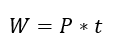## What is Energy:

Energy is nothing but the ability to do work. Also, the energy that must be transferred to do any small action. Example: if you want to through a ball for some distance, here you have spent some of your body energy. Energy is a quantitative property which must be transferred to substances or object to perform work. To perform a work or complete or to do something, energy should be used otherwise you cannot perform work. As per law of energy conversion the energy that cannot be created or destroyed, you can convert energy from one form into another form. (i.e: mechanical energy can be converted into electrical energy in Generator) here the output of your energy conversion is work.

Also see: Free Electron Theory

Example: You can see in your home on your fan or other home appliances, here when you switch your fan which starts rotating, where the electrical energy is converted into mechanical energy and due to this you will get forced air that is resultant work.

The SI unit of energy is the joule, which is the energy transferred to an object by the work of moving it a distance of 1 meter against a force of 1 Newton

Also see: Best gate MCQ

## What is Power:

The rate of change of energy is called power. Otherwise, the amount of energy transferred per unit time.  The SI unit of power is the joule per second (J/s), but commonly power is called as the watt in honor of James Watt. Also, Power often called HP (Horse Power). Both power unit watt and hp is having the relationship and that can be written as..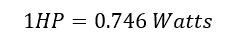Generally, Power is expressed as

As we Know I= Q/t, the rate of change of charge is equal to current. Apply this to above formula then we get..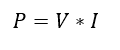Power can be derived as Multiple of Voltage and Current. This is the fundamental Power formula, this is mainly used in all electrical utility system. Unit of Power is Watt.

From above power equation, we can calculate energy expression.

Energy is nothing but a power consumed for the particular time.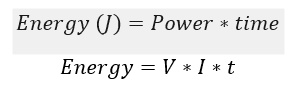This formula can be used in DC power sources, but in AC source there is an important factor must be added with the calculation, it is called as power factor.

Power factor is nothing but a cosine of the angle between the voltage and current.

In AC source energy consumption can be calculated as..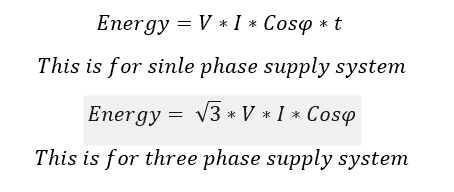Here Unit of energy is Watt-Hour